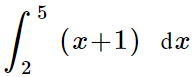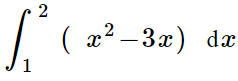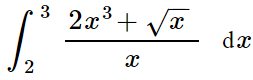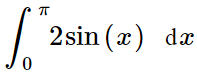### Day 54 - Fundamental Theorem of Calculus - 11.03.14

 UpdatesUnit 4 Test will be open note!Unit 4 Test will be on Friday, 11.14.14Bell RingerFundamental Theorem of CalculusWork on this solo! It will be counted as a quiz!Evaluate6.2513.530none of the aboveEvaluate13/6-13/627/6-27/6none of the aboveEvaluate6.226.613.30none of the aboveEvaluate0134none of the aboveReviewPrerequisitesDerivativesDerivative RulesPower RuleConstant RuleTrig DerivativesSigma NotationAntiderivativeIndefinite IntegralDefinitionIntegral SignIntegrandVariable of IntegrationConstant of IntegrationGeneral SolutionBasic Integration RulesArea Under a FunctionLessonFundamental Theorem of CalculusCheckpointsExit TicketPosted on the board at end of the block. Lesson Objective(s)How can the Fundamental Theorem of Calculus be used to solve problems?Standard(s)APC.10Use Riemann sums and the Trapezoidal Rule to approximate definite integrals of functions represented algebraically, graphically, and by a table of values and will interpret the definite integral as the accumulated rate of change of a quantity over an interval interpreted as the change of the quantity over the intervalRiemann sums will use left, right, and midpoint evaluation points over equal subdivisions.APC.11​The student will find antiderivatives directly from derivatives of basic functions and by substitution of variables (including change of limits for definite integrals).APC.12​The student will identify the properties of the definite integral. This will include additivity and linearity, the definite integral as an area, and the definite integral as a limit of a sum as well as the fundamental theorem.APC.13​The student will use the Fundamental Theorem of Calculus to evaluate definite integrals, represent a particular antiderivative, and facilitate the analytical and graphical analysis of functions so defined.APC.14​The student will find specific antiderivatives, using initial conditions (including applications to motion along a line). Separable differential equations will be solved and used in modeling (in particular, the equation y' = ky and exponential growth).APC.15​The student will use integration techniques and appropriate integrals to model physical, biological, and economic situations. The emphasis will be on using the integral of a rate of change to give accumulated change or on using the method of setting up an approximating Riemann sum and representing its limit as a definite integral. Specific applications will includea)​ the area of a region;b)​ the volume of a solid with known cross-section;c)​ the average value of a function; andd) ​the distance traveled by a particle along a line.Mathematical Practice(s)#1 - Make sense of problems and persevere in solving them#2 - Reason abstractly and quantitatively#5 - Use appropriate tools strategically#6 - Attend to precision#8 - Look for and express regularity in repeated reasoningPast CheckpointsAntiderivativeCheckpointsArea Under a FunctionCheckpoints